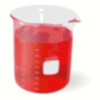#### You may also like### Plane to See

P is the midpoint of an edge of a cube and Q divides another edge in the ratio 1 to 4. Find the ratio of the volumes of the two pieces of the cube cut by a plane through PQ and a vertex.### 2D-3D

Two circles of equal size intersect and the centre of each circle is on the circumference of the other. What is the area of the intersection? Now imagine that the diagram represents two spheres of equal volume with the centre of each sphere on the surface of the other. What is the volume of intersection?### Brimful 2

Which of these infinitely deep vessels will eventually full up?

# Reach for Polydron

##### Age 16 to 18 Challenge Level:

This excellent solution came from Ruth from Manchester High School for Girls.

In the tetrahedron $ABCD$, let $ABC$ and $ACD$ be right angled. If you position the tetrahedron so that $ABC$ is the base, then the vertex $D$ is directly above the edge $AC$. This means that the height of the tetrahedron is the height of the triangle $ACD$ which is $\frac{1}{\sqrt{2}}$ and the area of the base of the tetrahedron is the area of the triangle $ABC$ which is $\frac{1}{2}$. The volume of a pyramid is one third base times height. Therefore

$$\begin{eqnarray} V &=& \frac{1}{3}\frac{1}{2} \frac{1}{\sqrt{2}} \\ &=&\frac{1}{6 \sqrt{2}} \\ &=& \frac{\sqrt{2}}{12} \end{eqnarray}$$